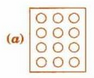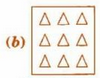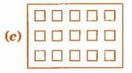Q

# Shade 1/2 of the circles in box (a) ,2/3 of the triangles in box (b), 3/5 of the squares in box (c)

of the circles in box (a)                   of the triangles in box (b)

of the squares in box (c).Views

1) In figure a there are 12 circles: half of 12 = 6

2) In figure b there are 9 triangles: 2/3 of 9 = 6

3) In figure c there are 15 triangles: 3/5 of 15 = 9Exams
Articles
Questions